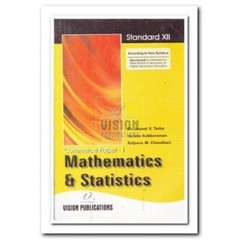# MATHEMATICS & STATISTICS P-I

(Inclusive of all Taxes)

Rs. 87

## Details

Contents:

1. Mathematical Logic
1.1 Introduction
1.2 Statements
1.3 Logical connectives
1.4 Statement pattern and logical equivalence
1.5 Statement forms and their diagrammatic representation
1.6 Negation of a Statement
1.7 Duality Theorem
2. Matrices
2.1 Introduction
2.2 definition
2.3 types of the matrices
2.4 algebra of matrices
2.5 Elementary Transformations or Operations
2.6 Effect of the elementary operations on product of the matrices
2.7 Inverse of the matrix
2.8 Solution of linear equations
3. Limits and Continuity
3.1 Introduction
3.2 Definition of limit
3.3 Limits of trignometric functions
3.5 Continuity of a function at a point
4. Differentiation (Derivative)
4.1 Introduction
4.2 Derivative at a point
4.3 Differentiation Rules
4.4 Derivative of A Composite Function
4.5 Derivative of Inverse Function
4.6 Logarithmic Differentiation
4.7 Derivative of Implicit Function
4.8 Derivative of Parametric Function
5. Applications of Derivatives
5.1 Introduction
5.2 Second Order Derivative (Notation)
5.3 Approximation and Errors
5.4 Increasing and Decreasing Functions
6. Integration
6.1 Introduction
6.2 Indefinite integral
6.3 Integration of Standard Functions
6.4 Rules of Integration
6.5 Methods of integration
6.6 Integration by Parts
6.7 Integration by partial fraction
7. Definite Integration
7.1 Introduction
7.2 Fundamental theorem of integral calculus
7.3 Properties of finite integrals
8. Differential Equation
8.1 Introduction
8.2 Solution of differential equation of first order and first degree 210
9. Application of Differential Equations
9.1 Introduction
9.2 Law of Growth and Decay
9.3 Population and Bacterial growth

• Phase Junior College

• Stream Commerce

• Standard/Year 12th

• Medium English

• Board/University Maharashtra State Board

• Subject Mathematics

#### Reviews

• No Reviews written for this product. Be the first to write a review.